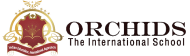Enquire Now

# Calculator

### Calculator

What is a Calculator?

A calculator is an electronic device that is used to do mathematical calculations. It performs arithmetic operations on numbers. Basic calculators can perform mathematical calculations such as addition, subtraction, multiplication, and division.

Calculators usually include an 8-to-12-digit display. There are also physical keys where students can manually enter the desired digit. Basic calculators usually run on batteries.

Why do we use Calculators?

To fast forward the calculation process and avoid errors is one of the basic reasons. Calculations can be stressful sometimes, and the calculator makes it reposeful for everyone. Calculators are simple and efficient devices for parents and students to solve complex operations. It is an essential tool for students.

The calculator allows students to save time from doing manual calculations. While using a calculator, students can do extensive calculations with accurate results in a short time.

Types of calculators

There are many types of calculators by which students can make the calculations easier. Here are some calculators which can be used to make calculations easier.

1. Basic Calculators
2. Math Calculators
3. Physics Calculators
4. Chemistry Calculators
List of Calculators
 School Age Calculator Child Education Calculator Marks Percentage Calculator Percentage Calculator Multiplication Calculator Division Calculator Addition Calculator Square Root Calculator Basic Calculator Discount Calculator Mass Calculator Power Calculator Wavelength Calculator Compound Interest Calculator

## FAQs

##### What is a scientific calculator?

| K12 Techno Services ®

ORCHIDS - The International School | Terms | Privacy Policy | Cancellation